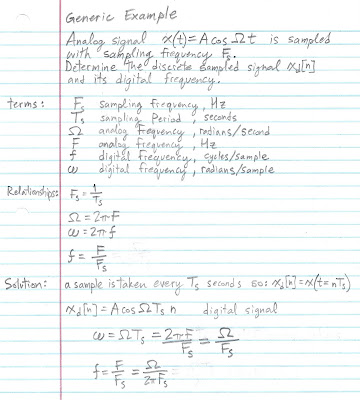Monday, March 22, 2010

Digital Frequency, Sampling a Sinusoid

I am preparing for an exam in DSP (EEE 404 at ASU) and I've been confused about digital frequency. It seems like a simple enough concept but I've been getting it mixed up. So as I sort it out I decided to blog it for my benefit and because I can't seem to find many examples. If I can post a decent blog about it then I figure I'm at a point where I understand it so here it goes.
Initially I learned to use a lowercase omega for angular frequency and a lowercase f for frequency (Hz). Then in this class they use capital omega for analog angular frequency, capital F for analog frequency, lowercase omega for digital angular frequency and lowercase f for digital frequency. This little symbol change has tripped me up. I wrote out the basics to help me keep it all straight:Note that n in the above example is restricted to integer values. Now that I have all that down it should be the easiest part of the test, but let's think about the meaning of digital frequency: I believe it can be thought of as the fraction of the continuous signal's cycle that is represented by a single sample. I say fraction because if you follow Nyquist's Sampling Theorem for signal recovery (simpler explanation of Nyquist theorem here), your sampling frequency should be at least twice the frequency of the signal frequency and noting that digital frequency is the ratio of frequency to sampling frequency it is evident that digital frequency will be less than 0.5. It is possible to neglect the sampling theorem and doing so shines the light upon an important difference between discrete samples of sinusoids and their continuous counterparts: For a sampled signal with f greater than 0.5 there exists a signal with f less than 0.5 which is the same!
In the next example I have sampled a continuous signal at two different frequencies, the first with f greater than 0.5 and the second with f less than 0.5. The continuous signal is with light dashed lines and the discrete samples are bold impulses, they share the same plot (the horizontal axis thus represents both t and n). Notice that the first set of discrete points suggests a sinusoid of a lower frequency (namely 1/4th of the original frequency) and therein lies the danger in having your sampling frequency too small.I think I've got a pretty good handle on this, now I need to study all the other stuff that may be on this exam.